# Problems of the Week

Contribute a problem

# 2018-07-02 Intermediate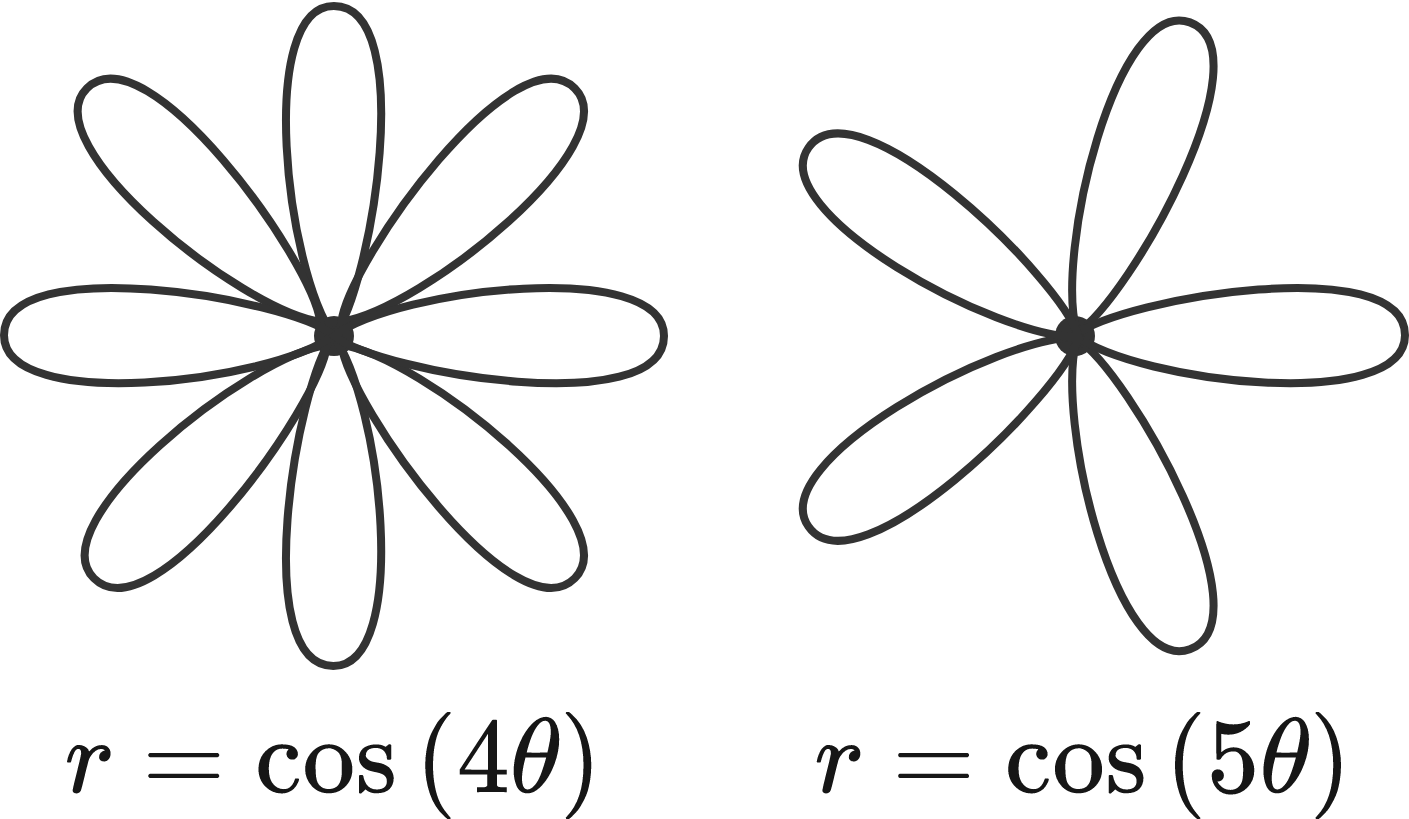$P(k)$ is the number of petals in a rose formed by the polar equation $r = \cos(k\theta).$

For example, $P(4) = 8$ because $r = \cos(4\theta)$ has $8$ petals, and $P(5) = 5$ because $r = \cos(5\theta)$ has $5$ petals.

Is there a positive integer $k$ such that $P(k) = 2018?$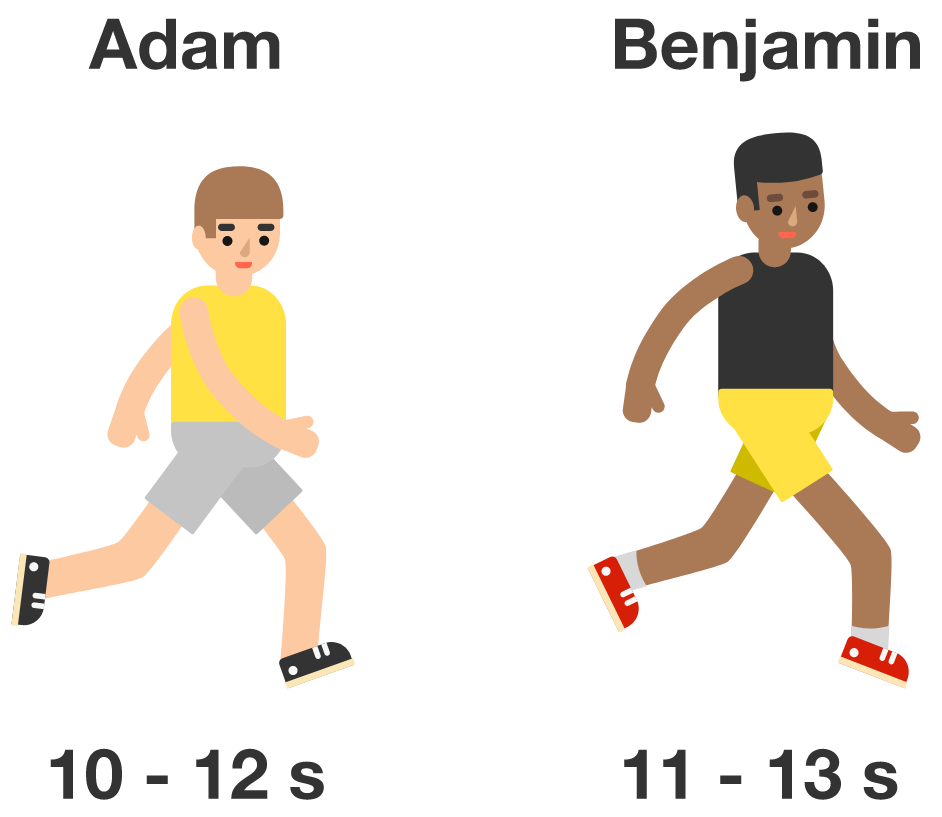For a 100-meter race, Adam’s race time ranges from 10-12 seconds, and Benjamin’s ranges from 11-13 seconds. Both times are independent and uniformly distributed.

If Adam and Benjamin run a 100-meter race together, what is the probability that Adam wins?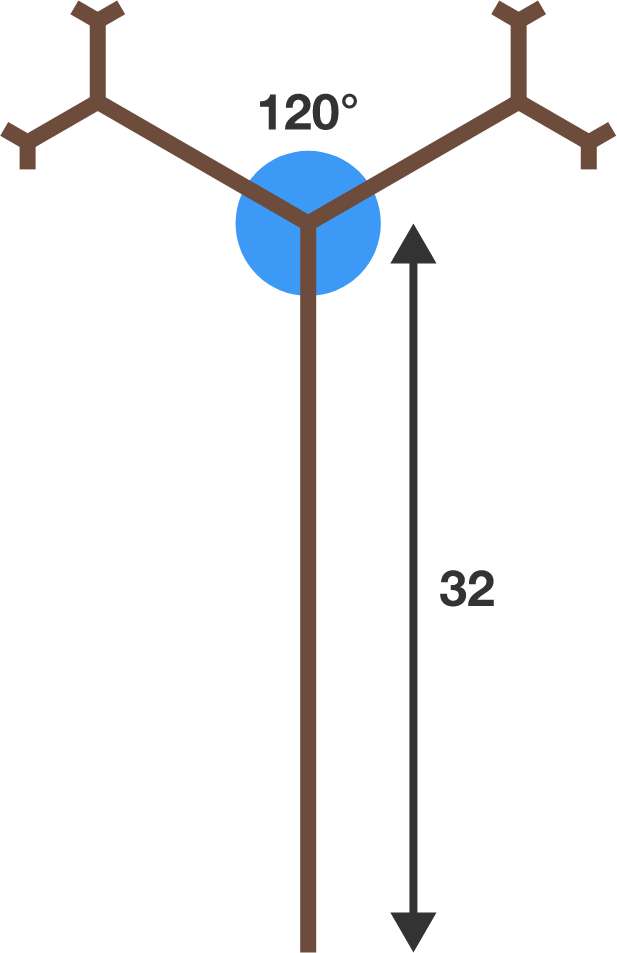A tree undergoes a sprouting growth.

Its longest branch is 32 units long, and the pair of branches connected to it are both $\frac{1}{3}$ the length of that branch. The same pattern continues with the length consistently shortening by $\frac{1}{3}$ in each step. Adjacent branches meet at $120^\circ.$

If this tree sprouts an infinite number of branches, what is its total height?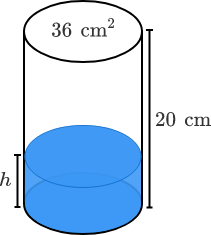A lot of skill goes into whether you can achieve a bottle flip. But with a bit of physics, you can maximize your chances by optimizing the volume of water in the bottle. Over- or under-filling the water bottle yields a high center of gravity, which can cause the bottle to tip over on landing, even if the throw is otherwise perfect. To stabilize the bottle's landing, the center of gravity should be as low as possible.

Treat an empty water bottle as a cylinder with mass $\SI{12}{\gram}$ evenly distributed along its length. What is the water level $h$ in centimeters such that the bottle's center of gravity is lowest?

Note: The density of water is about $\SI[per-mode=symbol]{1}{\gram \per \centi \meter \cubed}.$A light ray emitted from a vertex of this isosceles triangle reflects 6 times before reaching another vertex.

What is the total length of the path of this ray?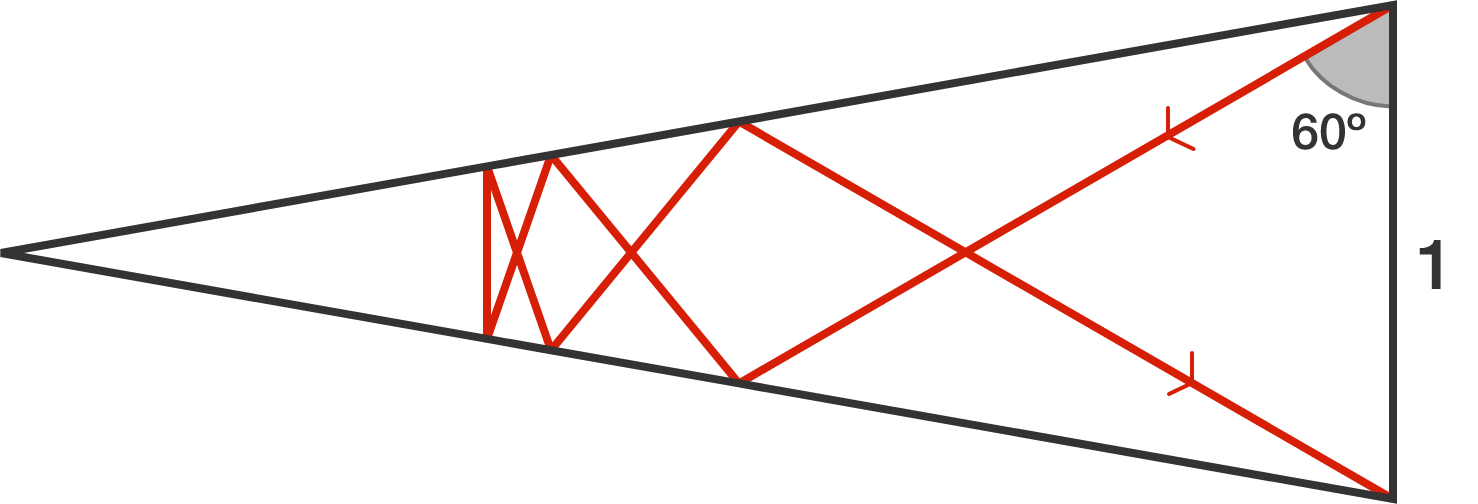×